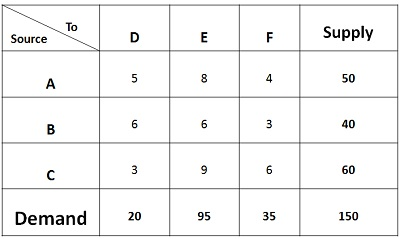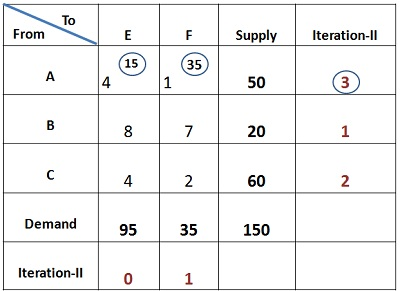# Different Types Methods for Finding Initial Solution by North – West Corner Rule, Least Cost Method and Vogal Approximation Method

### NORTH-WEST CORNER RULE

The North-West Corner Rule is a method adopted to compute the initial feasible solution of the transportation problem. The name North-west corner is given to this method because the basic variables are selected from the extreme left corner.

The concept of North-West Corner can be well understood through a transportation problem given below:In the table, three sources A, B and C with the production capacity of 50 units, 40 units, 60 units of product respectively is given. Every day the demand of three retailers D, E, F is to be furnished with at least 20 units, 95 units and 35 units of product respectively. The transportation costs are also given in the matrix.

The prerequisite condition for solving the transportation problem is that demand should be equal to the supply. In case the demand is more than supply, then dummy origin is added to the table. The supply of dummy origin will be equal to the difference between the total supply and total demand. The cost associated with the dummy origin will be zero.

Similarly, in case the supply is more than the demand, then dummy source is created whose demand will be equivalent to the difference between supply and demand. Again the cost associated with the dummy source will be zero.

Once the demand and supply are equal, the following procedure is followed:1. Select the north-west or extreme left corner of the matrix, assign as many units as possible to cell AD, within the supply and demand constraints. Such as 20 units are assigned to the first cell, that satisfies the demand of destination D while the supply is in surplus.
2. Now move horizontally and assign 30 units to the cell AE. Since 30 units are available with the source A, the supply gets fully saturated.
3. Now move vertically in the matrix and assign 40 units to Cell BE. The supply of source B also gets fully saturated.
4. Again move vertically, and assign 25 units to cell CE, the demand of destination E is fulfilled.
5. Move horizontally in the matrix and assign 35 units to cell CF, both the demand and supply of origin and destination gets saturated. Now the total cost can be computed.

The Total cost can be computed by multiplying the units assigned to each cell with the concerned transportation cost. Therefore,

Total Cost = 20*5+ 30*8+ 40*6+ 25*9+ 35*6 = Rs 1015

### LEAST COST METHOD

The Least Cost Method is another method used to obtain the initial feasible solution for the transportation problem. Here, the allocation begins with the cell which has the minimum cost. The lower cost cells are chosen over the higher-cost cell with the objective to have the least cost of transportation.

The Least Cost Method is considered to produce more optimal results than the North-west Corner because it considers the shipping cost while making the allocation, whereas the North-West corner method only considers the availability and supply requirement and allocation begin with the extreme left corner, irrespective of the shipping cost.

Let’s understand the concept of Least Cost method through a problem given below:In the given matrix, the supply of each source A, B, C is given Viz. 50units, 40 units, and 60 units respectively. The weekly demand for three retailers D, E, F i.e. 20 units, 95 units and 35 units is given respectively. The shipping cost is given for all the routes.

The minimum transportation cost can be obtained by following the steps given below:1. The minimum cost in the matrix is Rs 3, but there is a tie in the cell BF, and CD, now the question arises in which cell we shall allocate. Generally, the cost where maximum quantity can be assigned should be chosen to obtain the better initial solution. Therefore, 35 units shall be assigned to the cell BF. With this, the demand for retailer F gets fulfilled, and only 5 units are left with the source B.
2. Again the minimum cost in the matrix is Rs 3. Therefore, 20 units shall be assigned to the cell CD. With this, the demand of retailer D gets fulfilled. Only 40 units are left with the source C.
3. The next minimum cost is Rs 4, but however, the demand for F is completed, we will move to the next minimum cost which is 5. Again, the demand of D is completed. The next minimum cost is 6, and there is a tie between three cells. But however, no units can be assigned to the cells BD and CF as the demand for both the retailers D and F are saturated. So, we shall assign 5 units to Cell BE. With this, the supply of source B gets saturated.
4. The next minimum cost is 8, assign 50 units to the cell AE. The supply of source A gets saturated.
5. The next minimum cost is Rs 9; we shall assign 40 units to the cell CE. With his both the demand and supply of all the sources and origins gets saturated.

The total cost can be calculated by multiplying the assigned quantity with the concerned cost of the cell. Therefore,

Total Cost = 50*8 + 5*6 + 35*3 +20*3 +40*9 = Rs 955.

Note: The supply and demand should be equal and in case supply are more, the dummy source is added in the table with demand being equal to the difference between supply and demand, and the cost remains zero. Similarly, in case the demand is more than supply, then dummy destination or origin is added to the table with the supply equal to the difference in quantity demanded and supplied and the cost being zero.

VOGEL’S APPROXIMATION METHOD

Definition: The Vogel’s Approximation Method or VAM is an iterative procedure calculated to find out the initial feasible solution of the transportation problem. Like Least cost Method, here also the shipping cost is taken into consideration, but in a relative sense.

The following is the flow chart showing the steps involved in solving the transportation problem using the Vogel’s Approximation Method:The concept of Vogel’s Approximation Method can be well understood through an illustration given below:

• First of all the difference between two least cost cells are calculated for each row and column, which can be seen in the iteration given for each row and column. Then the largest difference is selected, which is 4 in this case. So, allocate 20 units to cell BD, since the minimum cost is to be chosen for the allocation. Now, only 20 units are left with the source B.• Column D is deleted, again the difference between the least cost cells is calculated for each row and column, as seen in the iteration below. The largest difference value comes to be 3, so allocate 35 units to cell AF and 15 units to the cell AE. With this, the Supply and demand of source A and origin F gets saturated, so delete both the row A and Column F.• Now, single column E is left, since no difference can be found out, so allocate 60 units to the cell CE and 20 units to cell BE, as only 20 units are left with source B. Hence the demand and supply are completely met.Now the total cost can be computed, by multiplying the units assigned to each cell with the cost concerned. Therefore,

Total Cost = 20*3 + 35*1 + 15*4 + 60*4 + 20*8 = Rs 555

Note: Vogel’s Approximation Method is also called as Penalty Method because the difference costs chosen are nothing but the penalties of not choosing the least cost routes.

## One thought on “Different Types Methods for Finding Initial Solution by North – West Corner Rule, Least Cost Method and Vogal Approximation Method”

error: Content is protected !!